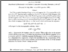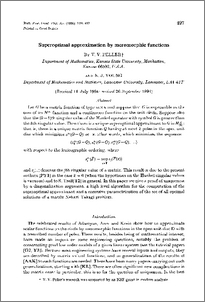# Super-optimal approximation by meromorphic functions.

Peller, V. V. and Young, N. J. (1996) Super-optimal approximation by meromorphic functions. Mathematical Proceedings of the Cambridge Philosophical Society, 119 (3). pp. 497-511. ISSN 0305-0041Preview

## Abstract

Let G be a matrix function of type m × n and suppose that G is expressible as the sum of an H∞ function and a continuous function on the unit circle. Suppose also that the (k – 1)th singular value of the Hankel operator with symbol G is greater than the kth singular value. Then there is a unique superoptimal approximant to G in : that is, there is a unique matrix function Q having at most k poles in the open unit disc which minimizes s∞(G – Q) or, in other words, which minimizes the sequence with respect to the lexicographic ordering, where and Sj(·) denotes the jth singular value of a matrix. This result is due to the present authors [PY1] in the case k = 0 (when the hypothesis on the Hankel singular values is vacuous) and to S. Treil[T2] in general. In this paper we give a proof of uniqueness by a diagonalization argument, a high level algorithm for the computation of the superoptimal approximant and a recursive parametrization of the set of all optimal solutions of a matrix Nehari—Takagi problem.

Item Type:
Journal Article
Journal or Publication Title:
Mathematical Proceedings of the Cambridge Philosophical Society
http://journals.cambridge.org/action/displayJournal?jid=PSP The final, definitive version of this article has been published in the Journal, Mathematical Proceedings of the Cambridge Philosophical Society, 119 (3), pp 497-511 1996, © 1996 Cambridge University Press.
Uncontrolled Keywords:
/dk/atira/pure/researchoutput/libraryofcongress/qa
Subjects:
ID Code:
19552
Deposited By:
Deposited On:
11 Nov 2008 12:59
Refereed?:
Yes
Published?:
Published